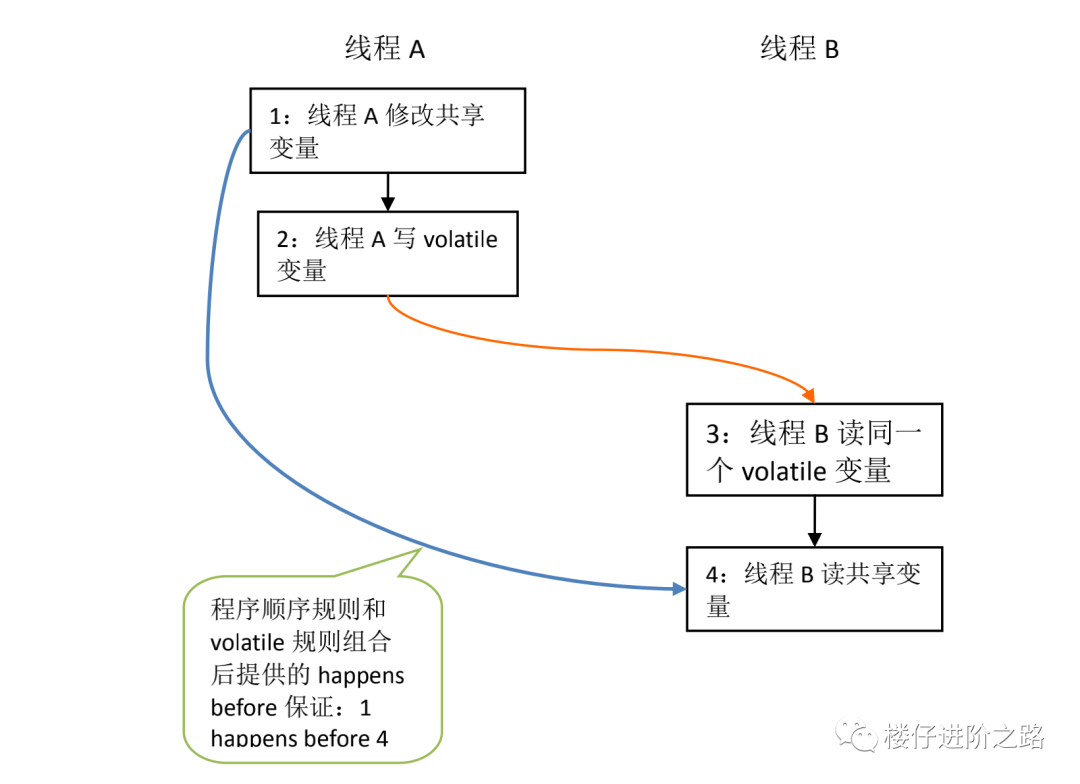2022年3月23日

# # Java并发编程volatile关键字解析

“三妹啊，这节我们来学习 Java 并发编程中的 volatile 关键字，以及容易遇到的坑。”看着三妹好学的样子，我倍感欣慰。

“好呀，哥。”三妹愉快的答应了。

## # volatile 变量的特性

volatile 可以保证可见性，但不保证原子性：

• 当写一个 volatile 变量时，JMM 会把该线程本地内存中的变量强制刷新到主内存中去；
• 这个写操作会导致其他线程中的 volatile 变量缓存无效。

## # volatile 禁止指令重排规则

• 重排序操作不会对存在数据依赖关系的操作进行重排序。比如：a=1;b=a; 这个指令序列，由于第二个操作依赖于第一个操作，所以在编译时和处理器运行时这两个操作不会被重排序。
• 重排序是为了优化性能，但是不管怎么重排序，单线程下程序的执行结果不能被改变。比如：a=1;b=2;c=a+b 这三个操作，第一步（a=1)和第二步(b=2)由于不存在数据依赖关系， 所以可能会发生重排序，但是 c=a+b 这个操作是不会被重排序的，因为需要保证最终的结果一定是 c=a+b=3。

• 当程序执行到 volatile 变量的读操作或者写操作时，在其前面的操作的更改肯定全部已经进行，且结果已经对后面的操作可见；在其后面的操作肯定还没有进行；
• 在进行指令优化时，不能将对 volatile 变量访问的语句放在其后面执行，也不能把 volatile 变量后面的语句放到其前面执行。

“二哥，能不能通俗地讲讲啊？”

“也就是说，执行到 volatile 变量时，其前面的所有语句都执行完，后面所有语句都未执行。且前面语句的结果对 volatile 变量及其后面语句可见。”我瞅了了三妹一眼说。

## # volatile 禁止指令重排分析

``````class ReorderExample {
int a = 0;
boolean flag = false;
public void writer() {
a = 1;                   //1
flag = true;             //2
}
Public void reader() {
if (flag) {                //3
int i =  a * a;        //4
System.out.println(i);
}
}
}
``````

``````class ReorderExample {
int a = 0;
boolean volatile flag = false;
public void writer() {
a = 1;                   //1
flag = true;             //2
}
Public void reader() {
if (flag) {                //3
int i =  a * a;        //4
System.out.println(i);
}
}
}
``````

1. 根据程序次序规则，1 happens before 2; 3 happens before 4。
2. 根据 volatile 规则，2 happens before 3。
3. 根据 happens before 的传递性规则，1 happens before 4。• 黑色箭头表示程序顺序规则；
• 橙色箭头表示 volatile 规则；
• 蓝色箭头表示组合这些规则后提供的 happens before 保证。

## # volatile 不适用场景

### # volatile 不适合复合操作

``````public class volatileTest {
public volatile int inc = 0;
public void increase() {
inc++;
}
public static void main(String[] args) {
final volatileTest test = new volatileTest();
for(int i=0;i<10;i++){
public void run() {
for(int j=0;j<1000;j++)
test.increase();
};
}.start();
}
System.out.println("inc output:" + test.inc);
}
}
``````

``````inc output:8182
``````

“为什么呀？二哥？”三妹疑惑地问。

“因为 inc++不是一个原子性操作，由读取、加、赋值 3 步组成，所以结果并不能达到 10000。”我耐心地回答。

“哦，你这样说我就理解了。”三妹点点头。

### # 解决方法

``````public class volatileTest1 {
public int inc = 0;
public synchronized void increase() {
inc++;
}
public static void main(String[] args) {
final volatileTest1 test = new volatileTest1();
for(int i=0;i<10;i++){
public void run() {
for(int j=0;j<1000;j++)
test.increase();
};
}.start();
}
System.out.println("add synchronized, inc output:" + test.inc);
}
}
``````

``````public class volatileTest2 {
public int inc = 0;
Lock lock = new ReentrantLock();
public void increase() {
lock.lock();
inc++;
lock.unlock();
}
public static void main(String[] args) {
final volatileTest2 test = new volatileTest2();
for(int i=0;i<10;i++){
public void run() {
for(int j=0;j<1000;j++)
test.increase();
};
}.start();
}
System.out.println("add lock, inc output:" + test.inc);
}
}
``````

``````public class volatileTest3 {
public AtomicInteger inc = new AtomicInteger();
public void increase() {
inc.getAndIncrement();
}
public static void main(String[] args) {
final volatileTest3 test = new volatileTest3();
for(int i=0;i<10;i++){
public void run() {
for(int j=0;j<100;j++)
test.increase();
};
}.start();
}
System.out.println("add AtomicInteger, inc output:" + test.inc);
}
}
``````

``````add synchronized, inc output:1000
add lock, inc output:1000
add AtomicInteger, inc output:1000
``````

## # 单例模式的双重锁要加volatile

``````public class penguin {
private static volatile penguin m_penguin = null;
// 避免通过new初始化对象
private void penguin() {}
public void beating() {
System.out.println("打豆豆");
};
public static penguin getInstance() {      //1
if (null == m_penguin) {               //2
synchronized(penguin.class) {      //3
if (null == m_penguin) {       //4
m_penguin = new penguin(); //5
}
}
}
return m_penguin;                      //6
}
}
``````

``````a. memory = allocate() //分配内存
b. ctorInstanc(memory) //初始化对象
c. instance = memory   //设置instance指向刚分配的地址
``````

## # 总结

“好了，三妹，我们来总结一下。”我舒了一口气说。

volatile 可以保证线程可见性且提供了一定的有序性，但是无法保证原子性。在 JVM 底层 volatile 是采用“内存屏障”来实现的。

• 它确保指令重排序时不会把其后面的指令排到内存屏障之前的位置，也不会把前面的指令排到内存屏障的后面；即在执行到内存屏障这句指令时，在它前面的操作已经全部完成；
• 它会强制将对缓存的修改操作立即写入主存；
• 如果是写操作，它会导致其他 CPU 中对应的缓存行无效。